# Exponential Regression - calculate with Matlab

We’ll work this time with exponential regression in a curve fitting example. The following codes find the coefficients of an equation for an exponential curve.

 The equation is in the following form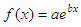where a and b are the calculated coefficients. The equation coefficients, coefficient of determination, coefficient of correlation and standard error of estimate are also displayed.

You must provide the x and y coordinates for known data points. Once the curve has been fitted you may predict values of y = f(x) for given values of x.

We’re going to experiment with three different methods to cope with our exponential regression.

The first method is a classical computation using known formulas. The second method deals with strategic optimization techniques and gives another example of the simplex method implemented by the Nelder-Mead algorithm used in the Matlab function fminsearch. The third method just uses interpolation capabilities in Matlab, it doesn’t calculate any coefficient, but can solve interpolation values, and can even extrapolate to a certain degree.

Example:

The table below shows the number of strange particles present in a scientific experiment at various points in time. We have to fit an exponential curve to the data and estimate the number of particles after 7 hours.

number of hours = [0 1 2 3 4 5 6]
number of particles = [25 38 58 89 135 206 315]

### First method: using known formulas

We know that coefficients a and b are determined by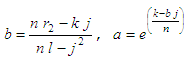where

n = number of points in given data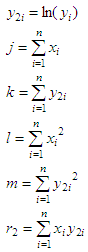We also know that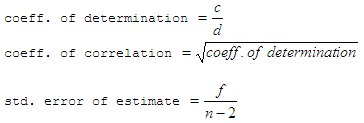where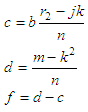Then, this is easy to code...

clear, clc, close all, format compact, format long

% enter data
x = 0 : 6;
y = [25 38 58 89 135 206 315];

% calculate coefficients a and b
n = length(x);
y2 = log(y);
j = sum(x);
k = sum(y2);
l = sum(x.^2);
m = sum(y2.^2);
r2 = sum(x .* y2);
b = (n * r2 - k * j) / (n * l - j^2)
a = exp((k-b*j)/n)

% calculate coeff. of determination, coeff. of correlation
% and standard error of estimate

c = b * (r2 - j * k / n);
d = m - k^2 / n;
f = d - c;
cf_dt = c/d
corr = sqrt(cf_dt)
std_err = sqrt(f / (n - 2))

% We can calculate any point along the curve
x = input('Interpolation: ');
y = a * exp(b * x)

The results are:

b = 0.42237507956752
a = 24.96166337377739
cf_dt = 0.99999355156242
corr = 0.99999677577601
std_err = 0.00253817142571

Interpolation: 7
y = 4.800867130774966e+002

### Second method: optimization techniques with fminsearch

This is a very powerful technique that can be applied in curve fitting, exponential regressions, or nonlinear problems in general. We can obtain the coefficients without knowing the formulas above!

What we do is set the problem in such a way that it can be minimized. The term ‘minimization’ means that we’re comparing our guesses against the given data. The least error, after several automatic iterations, is going to be the solution to our problem. Matlab built-in function fminsearch tries different coefficients and stops when the least error has been found.

Let’s say that we prepare this objective-function:

function U = OF_exp_regr(c)
global x y

% try coefficients
y2 = c(1) * exp(c(2) * x);

% try to match original data, and return difference
U = norm(y2 - y, 1);

We can now use that objective with the following code:

clear, clc, close all, format compact
global x y

% enter data and plot it
x = 0 : 6;
y = [25 38 58 89 135 206 315];
plot(x, y,
'g-o')
hold
on

% enter starting point: before optimization
c0 = [1 1];
y0 = c0(1) * exp(c0(2) * x);
plot(x, y0,
'r-o')
legend(
'measured data',  'initial guess')

% launch optimization method
fx = 'OF_exp_regr';
[c, f, EF, out] = fminsearch(fx, c0)
yf = c(1) * exp(c(2) * x);

% plot results after optimization
figure
plot(x, y,
'g-o', x, yf, 'bo')
legend(
'measured data', 'final fit')

The results are: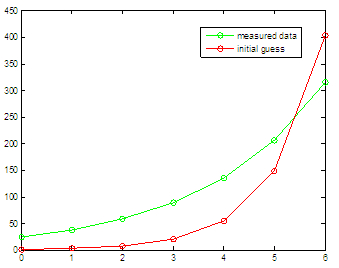c =  24.88778378033923   0.42303260089823
f =  1.08673354178606
EF = 1
out = iterations: 122
funcCount: 226You see? We started with a = 1 and b = 1 (as in c0 = [1 1]), and we got the initial graphic. The green line is the target, the red line is our initial guess. After the optimization process we got c(1)= a = 24.8877 and c(2)= b = 0.4230, and those coefficients produced the second plot, with green target and blue points showing our fit. The results are quite similar to those found by the method using formulas, right? It works!

### Third method: inter/extrapolation

This method doesn’t provide results for coefficients, but it an example of interpolation and extrapolation capabilities in Matlab.

We’ll use buil-in function interp1, whose basic syntax is:

yi = interp11(X, Y, XI, METHOD, 'extrap') uses the specified method for
extrapolation for any elements of XI outside the interval spanned by X.

Methods, among others, can be
'spline' piecewise cubic spline interpolation
'cubic'  shape-preserving piecewise cubic interpolation

The following code

clear, clc, close all, format compact

% plot initial data
x = 0 : 6;
y = [25 38 58 89 135 206 315];
plot(x, y,
'g-.o')
hold
on

% add more points using the coeff. obtained before
xi = [1.25 3.2 5.7 7 9];
a = 24.961663;
b = 0.422375;
ye = a * exp(b * xi);
plot(xi, ye,
'*g')

% inter/extrapolate with 'cubic' method
yi = interp1(x, y, xi, 'cubic', 'extrap');
plot(xi, yi,
'ro')

% inter/extrapolate with 'spline' method
yi = interp1(x, y, xi, 'spline', 'extrap');
plot(xi, yi,
'bo')
legend(
'Original data', 'Extra data', 'Cubic', 'Spline')

produces this plot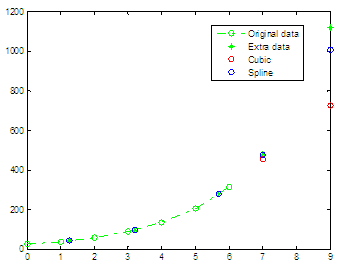we can see that interpolations work pretty well, but extrapolations diverge from the expected results. We can see that a spline method works better than a cubic one in this case, just for extrapolations.

From 'Exponential Regression' to home

From 'Exponential Regression' to 'Matlab Programming'